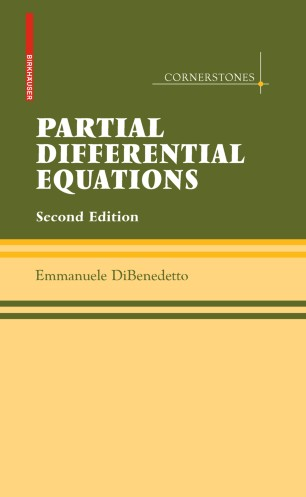# Partial Differential Equations

## Second Edition

• Emmanuele DiBenedettoTextbook

Part of the Cornerstones book series (COR)

1. Front Matter
Pages 1-20
2. Emmanuele DiBenedetto
Pages 1-16
3. Emmanuele DiBenedetto
Pages 17-35
4. Emmanuele DiBenedetto
Pages 37-86
5. Emmanuele DiBenedetto
Pages 87-107
6. Emmanuele DiBenedetto
Pages 109-134
7. Emmanuele DiBenedetto
Pages 135-181
8. Emmanuele DiBenedetto
Pages 183-224
9. Emmanuele DiBenedetto
Pages 225-263
10. Emmanuele DiBenedetto
Pages 265-295
11. Emmanuele DiBenedetto
Pages 297-345
12. Emmanuele DiBenedetto
Pages 347-371
13. Back Matter
Pages 1-17

### Introduction

This self-contained textbook offers an elementary introduction to partial differential equations (PDEs), primarily focusing on linear equations, but also providing a perspective on nonlinear equations, through Hamilton--Jacobi equations, elliptic equations with measurable coefficients and DeGiorgi classes. The exposition is complemented by examples, problems, and solutions that enhance understanding and explore related directions.

Large parts of this revised second edition have been streamlined and rewritten to incorporate years of classroom feedback, correct misprints, and improve clarity. The work can serve as a text for advanced undergraduates and graduate students in mathematics, physics, engineering, and the natural sciences, as well as an excellent reference for applied mathematicians and mathematical physicists.

The newly added three last chapters, on first order non-linear PDEs (Chapter 8), quasilinear elliptic equations with measurable coefficients (Chapter 9) and DeGiorgi classes (Chapter 10), point to issues and directions at the forefront of current investigations.

Reviews of the first edition:

The author's intent is to present an elementary introduction to PDEs... In contrast to other elementary textbooks on PDEs . . . much more material is presented on the three basic equations: Laplace's equation, the heat and wave equations. . . . The presentation is clear and well organized. . . . The text is complemented by numerous exercises and hints to proofs.

---Mathematical Reviews

This is a well-written, self-contained, elementary introduction to linear, partial differential equations.

---Zentralblatt MATH

### Keywords

Boundary value problem Cauchy--Kowalewski Theorem Conservation Laws Degiorgi Classes Elliptic Theory Green's Theorem Linear Elliptic Equations Partial Differential Equations Potential Viscosity Solutions partial differential equation wave equation

#### Authors and affiliations

• Emmanuele DiBenedetto
• 1
1. 1.Dept. MathematicsVanderbilt UniversityNashvilleU.S.A.

Industry Sectors